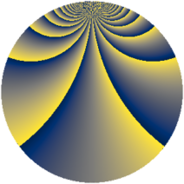# Properties

 Label 1344.4.cjLevel $1344$ Weight $4$ Character orbit 1344.cj Rep. character $\chi_{1344}(125,\cdot)$ Character field $\Q(\zeta_{16})$ Dimension $6112$ Sturm bound $1024$

# Related objects

## Defining parameters

 Level: $$N$$ $$=$$ $$1344 = 2^{6} \cdot 3 \cdot 7$$ Weight: $$k$$ $$=$$ $$4$$ Character orbit: $$[\chi]$$ $$=$$ 1344.cj (of order $$16$$ and degree $$8$$) Character conductor: $$\operatorname{cond}(\chi)$$ $$=$$ $$1344$$ Character field: $$\Q(\zeta_{16})$$ Sturm bound: $$1024$$

## Dimensions

The following table gives the dimensions of various subspaces of $$M_{4}(1344, [\chi])$$.

Total New Old
Modular forms 6176 6176 0
Cusp forms 6112 6112 0
Eisenstein series 64 64 0

## Trace form

 $$6112q - 32q^{4} - 16q^{7} - 16q^{9} + O(q^{10})$$ $$6112q - 32q^{4} - 16q^{7} - 16q^{9} - 16q^{15} - 32q^{16} - 16q^{18} - 8q^{21} - 32q^{22} - 32q^{25} - 16q^{28} - 2336q^{30} - 896q^{36} - 32q^{37} - 16q^{39} - 3168q^{42} - 32q^{43} - 32q^{46} - 16q^{49} - 16q^{51} - 16q^{57} - 32q^{58} - 16q^{60} - 16q^{63} + 12064q^{64} + 6496q^{67} - 16q^{70} - 16q^{72} - 16q^{78} - 32q^{79} - 16q^{81} - 4152q^{84} - 32q^{85} - 32q^{88} - 16q^{91} - 448q^{93} - 16q^{99} + O(q^{100})$$

## Decomposition of $$S_{4}^{\mathrm{new}}(1344, [\chi])$$ into newform subspaces

The newforms in this space have not yet been added to the LMFDB.